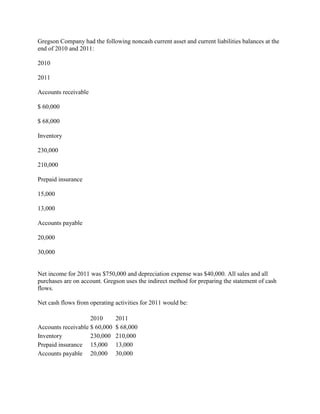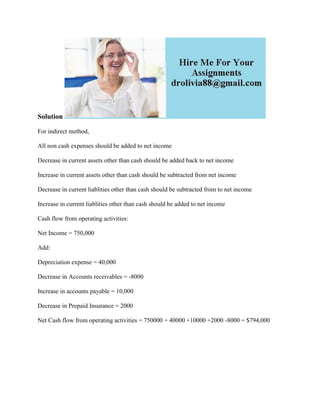Seu SlideShare está sendo baixado. ×

# Gregson Company had the following noncash current asset and current li.docx

Anúncio
Anúncio
Anúncio
Anúncio
Anúncio
Anúncio
Anúncio
Anúncio
Anúncio
Anúncio
AnúncioCarregando em…3
×

1 de 3 Anúncio

# Gregson Company had the following noncash current asset and current li.docx

Gregson Company had the following noncash current asset and current liabilities balances at the end of 2010 and 2011:
2010
2011
Accounts receivable
\$ 60,000
\$ 68,000
Inventory
230,000
210,000
Prepaid insurance
15,000
13,000
Accounts payable
20,000
30,000
Net income for 2011 was \$750,000 and depreciation expense was \$40,000. All sales and all purchases are on account. Gregson uses the indirect method for preparing the statement of cash flows.

Net cash flows from operating activities for 2011 would be:
Solution
For indirect method,
All non cash expenses should be added to net income
Decrease in current assets other than cash should be added back to net income
Increase in current assets other than cash should be subtracted from net income
Decrease in current liablities other than cash should be subtracted from to net income
Increase in current liablities other than cash should be added to net income
Cash flow from operating activities:
Net Income = 750,000
Depreciation expense = 40,000
Decrease in Accounts receivables = -8000
Increase in accounts payable = 10,000
Decrease in Prepaid Insurance = 2000
Net Cash flow from operating activities = 750000 + 40000 +10000 +2000 -8000 = \$794,000
.

Gregson Company had the following noncash current asset and current liabilities balances at the end of 2010 and 2011:
2010
2011
Accounts receivable
\$ 60,000
\$ 68,000
Inventory
230,000
210,000
Prepaid insurance
15,000
13,000
Accounts payable
20,000
30,000
Net income for 2011 was \$750,000 and depreciation expense was \$40,000. All sales and all purchases are on account. Gregson uses the indirect method for preparing the statement of cash flows.

Net cash flows from operating activities for 2011 would be:
Solution
For indirect method,
All non cash expenses should be added to net income
Decrease in current assets other than cash should be added back to net income
Increase in current assets other than cash should be subtracted from net income
Decrease in current liablities other than cash should be subtracted from to net income
Increase in current liablities other than cash should be added to net income
Cash flow from operating activities:
Net Income = 750,000
Depreciation expense = 40,000
Decrease in Accounts receivables = -8000
Increase in accounts payable = 10,000
Decrease in Prepaid Insurance = 2000
Net Cash flow from operating activities = 750000 + 40000 +10000 +2000 -8000 = \$794,000
.

Anúncio
Anúncio

### Gregson Company had the following noncash current asset and current li.docx

1. 1. Gregson Company had the following noncash current asset and current liabilities balances at the end of 2010 and 2011: 2010 2011 Accounts receivable \$ 60,000 \$ 68,000 Inventory 230,000 210,000 Prepaid insurance 15,000 13,000 Accounts payable 20,000 30,000 Net income for 2011 was \$750,000 and depreciation expense was \$40,000. All sales and all purchases are on account. Gregson uses the indirect method for preparing the statement of cash flows. Net cash flows from operating activities for 2011 would be: 2010 2011 Accounts receivable \$ 60,000 \$ 68,000 Inventory 230,000 210,000 Prepaid insurance 15,000 13,000 Accounts payable 20,000 30,000
2. 2. Solution For indirect method, All non cash expenses should be added to net income Decrease in current assets other than cash should be added back to net income Increase in current assets other than cash should be subtracted from net income Decrease in current liablities other than cash should be subtracted from to net income Increase in current liablities other than cash should be added to net income Cash flow from operating activities: Net Income = 750,000 Add: Depreciation expense = 40,000 Decrease in Accounts receivables = -8000 Increase in accounts payable = 10,000 Decrease in Prepaid Insurance = 2000 Net Cash flow from operating activities = 750000 + 40000 +10000 +2000 -8000 = \$794,000